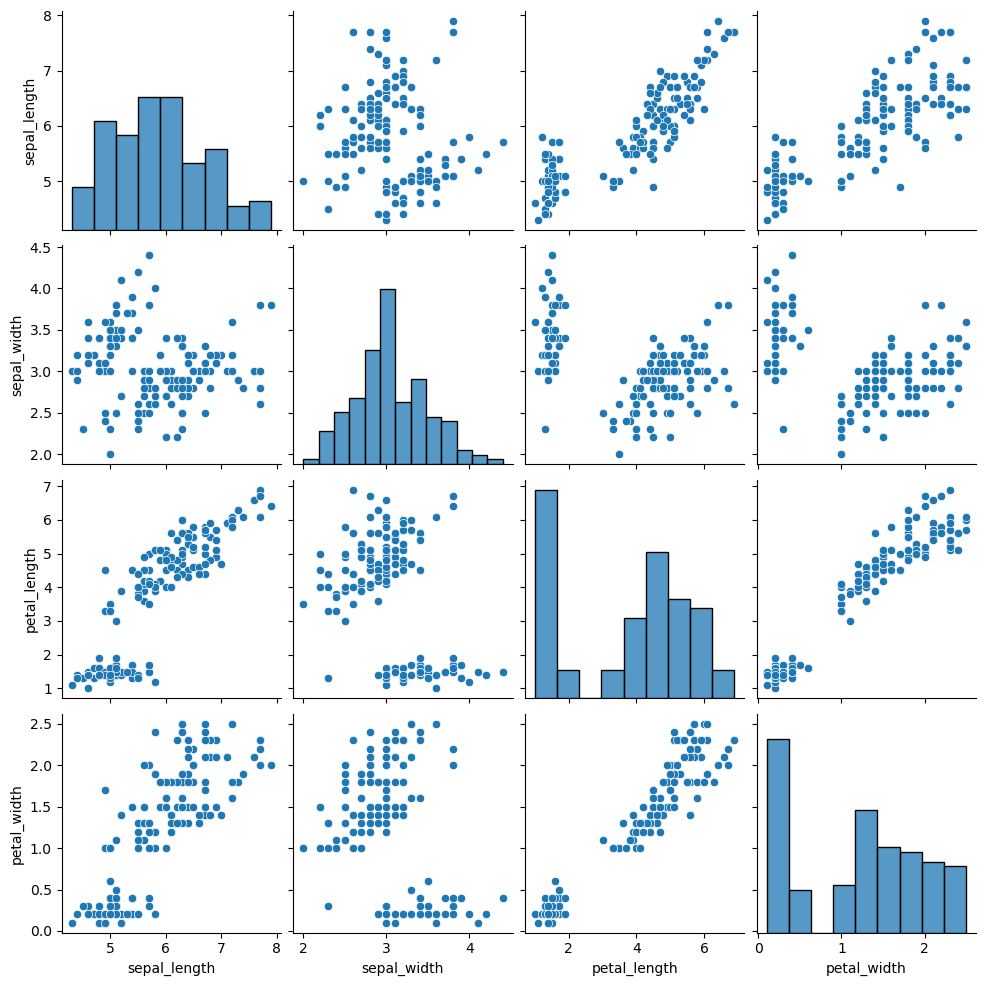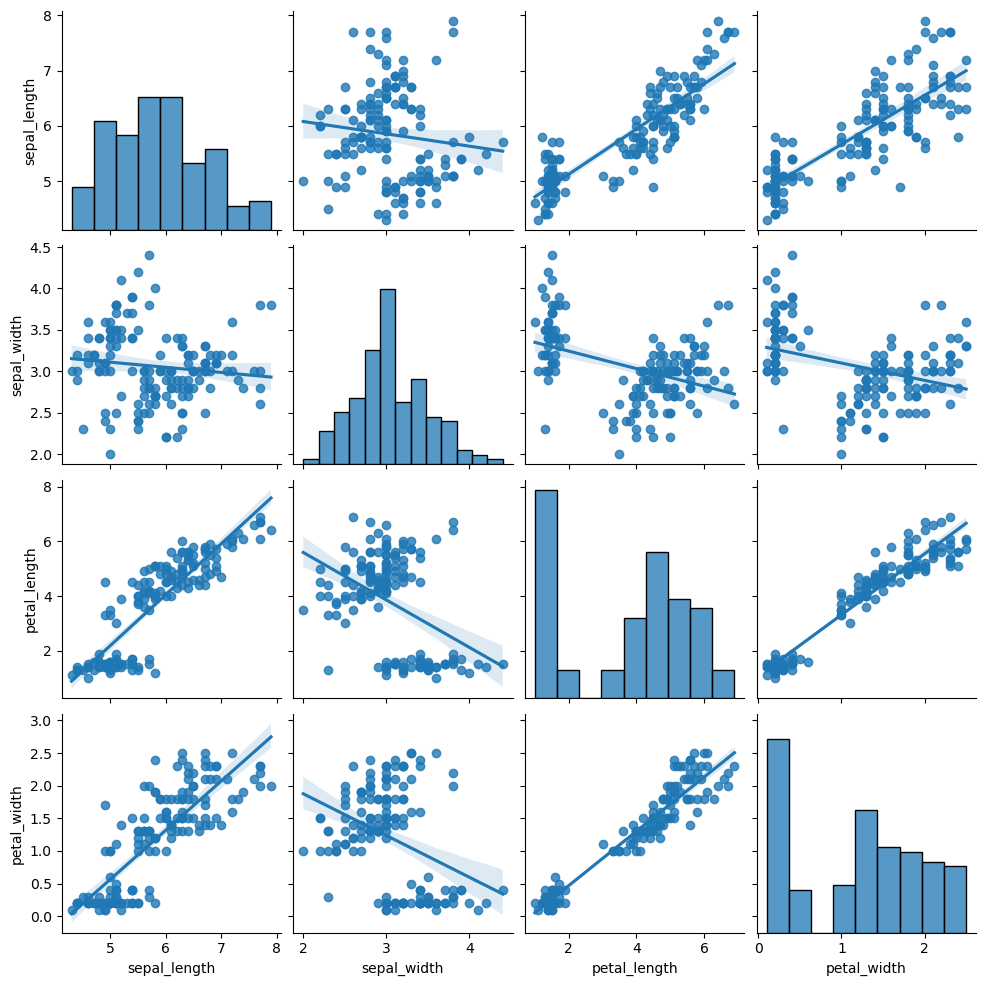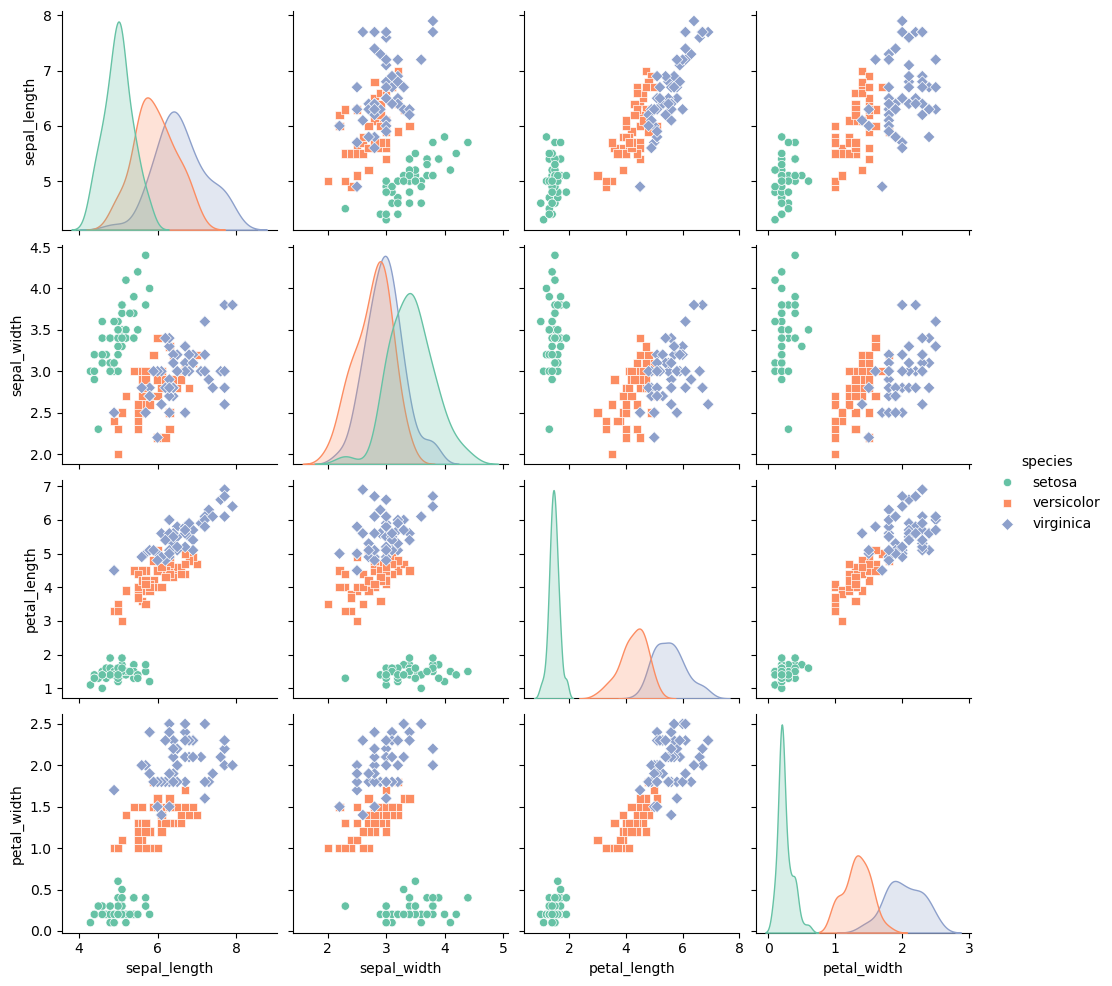# Definition

A `correlogram` or correlation matrix allows to analyse the relationship between each pair of numeric variables of a dataset. The relationship between each pair of variable is visualised through a scatterplot, or a symbol that represents the correlation (bubble, line, number..).

The diagonal often represents the `distribution` of each variable, using an histogram or a density plot.

``````# library & dataset
import seaborn as sns
import matplotlib.pyplot as plt

# Basic correlogram
sns_plot = sns.pairplot(df)
sns_plot.savefig("IMG/correlogram1.png")``````Note: exceptionally, graphic provided in this page are made with Python, since I really like the `pairplot` function of the seaborn library.

# What for

Correlogram are really handy for `exploratory analysis`. It allows to visualize the relationships of the whole dataset in a glimpse. For instance, the linear relationship between petal length and petal width is obvious here, as the one concerning sepal.

When you get a multivariate dataset, building a correlogram is one of the first step you should follow.

# Variation

All the variations described in the scatterplot section are also available for correlogram. For example, why not applying a linear regression to each pair of variable:

``````# with regression
sns_plot = sns.pairplot(df, kind="reg")
sns_plot.savefig("IMG/correlogram2.png")``````As described in the scatterplot section, it is a good practice to display subgroups if a categoric variable is available as well:

``````# with regression
sns_plot = sns.pairplot(df, kind="scatter", hue="species", markers=["o", "s", "D"], palette="Set2")
sns_plot.savefig("IMG/correlogram3.png")``````# Common mistakes

• Displaying the relationship between more than ~10 variables makes the plot very hard to read
• All the common caveats of scatterplot and histogram apply

# Build your own

The R and Python graph galleries are 2 websites providing hundreds of chart example, always providing the reproducible code. Click the button below to see how to build the chart you need with your favorite programing language.